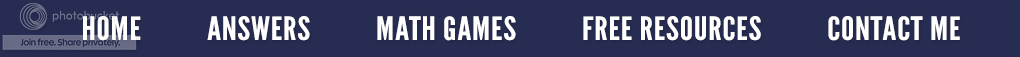I have decided to post (this is an updated previous post from 2011) some questions about zero that my college students have asked me in class.  I will say this, "Zero can surely give you a severe headache unless one knows its properties."

Question #1 - Do you know why zero is an even number?    All mathematics is based on patterns.  Because of this, I know that an even plus an even number will always give me an even answer; an odd number added to an odd also gives me an even answer, and an odd number plus an even gives me an odd answer. In other words:    E + E = E     O + O = E     O + E = O﻿﻿﻿﻿﻿﻿﻿
﻿﻿﻿﻿﻿﻿﻿
The numbers 4 and -4 are both even numbers. If we add them together, their sum is zero.  Based on the math pattern of  E + E = E,  then zero has to be even as well.  If we substitute zero in other problems such as 1 + 0 = 1, it fits the O + E = O  rule just as 2 + 0 = 2 fits the E + E = E  rule.

In Algebra, even numbers can be written as 2 x n where n is an integer.  Odd numbers can be written in the form of  2 x n + 1.  If we have n represent 0, then  2 x n = 0 (even) and  2 x n + 1 = 1. (odd)

﻿﻿I say all of this to relate an actual incident that occurred in my classroom.  I wrote the number 934 on the white board, and commented that since it was even it was divisible by 2.  One of my students was perplexed because he did not understand how 934 could be even when it contained two odd numbers and only one even number.  He actually thought that all the digits of a number had to be even for the number to be even.  Funny?  Not really!  Amazingly, he had made it through 12 years of school without understanding Place value as it relates to even numbers. Unfortunately, I had assumed that everyone (especially my college students) knew what an even number was.  I no longer make assumptions about students and their math knowledge!

Question #2 - Is zero positive or negative? The definition for positive numbers is all numbers greater than zero, and the definition for negative numbers is all numbers less than zero. Therefore zero can be neither positive or negative.

Question #3 - Is zero a prime or composite number? To be a prime number, a number must have only two positive divisors, itself and one. Zero has an infinite number of divisors so it is not prime. A composite number can be written as a product of two factors, neither of which is itself. Since zero cannot be written as a product of two factors without including itself, zero, it is not composite.

Question #4 - Why can't you divide by zero? I love this question. Back in the dark ages when I asked it, I was always told, "Because I said so." Being an inquisitive student was not a blessing when I was growing up. Math teachers who knew all did not want to be questioned!!!! Anyway, I don't mind the question, and here is my practical answer.

First, we must understand division. Division means putting or separating a number of items into a number of specific groups or sets. When you divide, such as in the problem 12 divided by 2, you are really putting 12 things into two groups or two sets. Therefore, if you have the problem 8 divided by 0, it is impossible to put eight things into no groups. You cannot put something into nothing!
﻿﻿
Hopefully, this clears up a few things about zero.  I leave you with this math cheer.  (I always wanted to be a cheerleader!)

Zero, two, four, six, eight,

Even numbers! Even Numbers! Even Numbers!